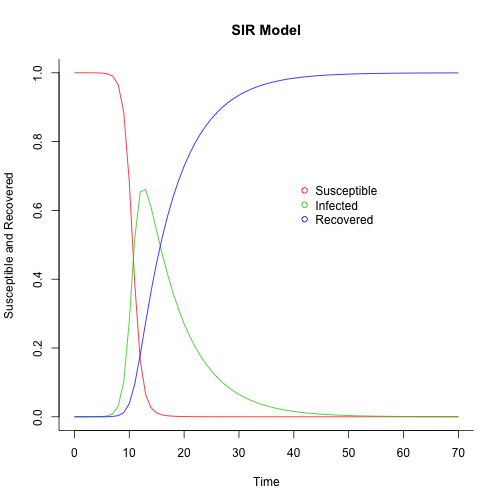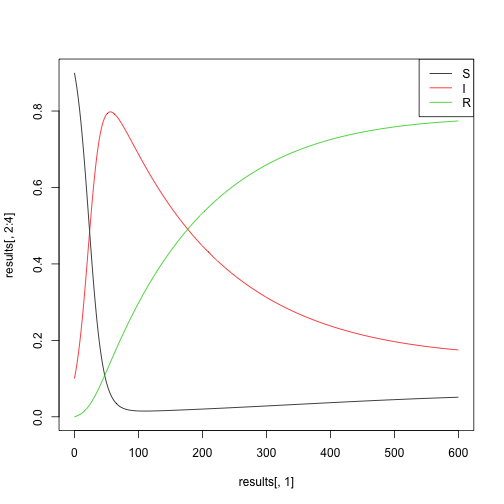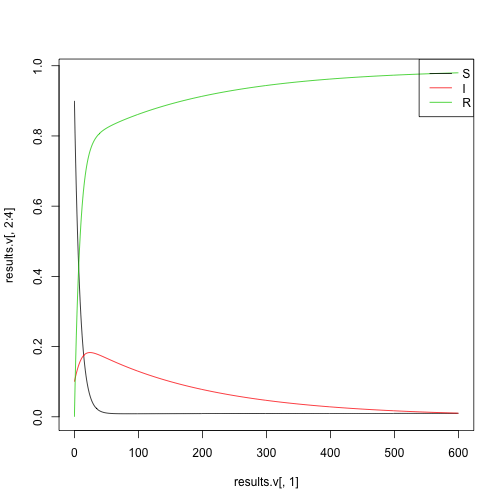# SIR models in R

## Demo by Dr Aidan Findlater (slightly modified)

This example is originally in Modeling Infectious Diseases in Humans and Animals: http://homepages.warwick.ac.uk/~masfz/ModelingInfectiousDiseases/index.html

``````## Load deSolve package
library(deSolve)

## Create an SIR function
sir <- function(time, state, parameters) {

with(as.list(c(state, parameters)), {

dS <- -beta * S * I
dI <-  beta * S * I - gamma * I
dR <-                 gamma * I

return(list(c(dS, dI, dR)))
})
}

### Set parameters
## Proportion in each compartment: Susceptible 0.999999, Infected 0.000001, Recovered 0
init       <- c(S = 1-1e-6, I = 1e-6, R = 0.0)
## beta: infection parameter; gamma: recovery parameter
parameters <- c(beta = 1.4247, gamma = 0.14286)
## Time frame
times      <- seq(0, 70, by = 1)

## Solve using ode (General Solver for Ordinary Differential Equations)
out <- ode(y = init, times = times, func = sir, parms = parameters)
## change to data frame
out <- as.data.frame(out)
## Delete time variable
out\$time <- NULL
## Show data
``````
``````        S           I            R
1  1.0000 0.000001000 0.0000000000
2  1.0000 0.000003968 0.0000003308
3  1.0000 0.000014339 0.0000014866
4  0.9999 0.000052804 0.0000057737
5  0.9998 0.000192686 0.0000213659
6  0.9992 0.000699082 0.0000778350
7  0.9972 0.002516429 0.0002807825
8  0.9900 0.009000750 0.0010086591
9  0.9649 0.031522809 0.0035835022
10 0.8846 0.103084442 0.0122934382
``````
``````
## Plot
matplot(x = times, y = out, type = "l",
xlab = "Time", ylab = "Susceptible and Recovered", main = "SIR Model",
lwd = 1, lty = 1, bty = "l", col = 2:4)

legend(40, 0.7, c("Susceptible", "Infected", "Recovered"), pch = 1, col = 2:4, bty = "n")
``````# HSPH EPI 203 examples

The code here is originally by Dr Caroline Buckee and colleagues. I have modified it slightly.

## Basic SIR model

``````## Define as a function
epi203 <- function(pars){

## Show parameters
print(pars)

times <- seq(from = 0, to = 600, by = 1)              # we want to run the model for 3000 time steps
yinit <- c(Susc = 0.9, Infected = 0.1, Recovered = 0) # this parameter sets the initial conditions

## below is the code for the actual model including the equations that you should recognize
SIR_model <- function(times, yinit, pars){

with(as.list(c(yinit,pars)), {

dSusc      <- birth - beta*Infected*Susc                     - death*Susc
dInfected  <-         beta*Infected*Susc - recovery*Infected - death*Infected
dRecovered <-                              recovery*Infected - death*Recovered

return(list(c(dSusc, dInfected, dRecovered)))})
}

## run the ode solver for the function specified (function defined above is used)
## return the value of each compartment (Susc, Infected, Recovered) for each time step.
results <- ode(func = SIR_model, times = times, y = yinit, parms = pars)
results <- as.data.frame(results)

## Return result
return(results)
}

##############################################################################

test.pars <- c(beta = 0.1, recovery = 0.005, death = 0.001, birth = 0.001)
results   <- epi203(test.pars)
``````
``````    beta recovery    death    birth
0.100    0.005    0.001    0.001
``````
``````
##############################################################################
## Plotting
matplot(results[, 1], results[, 2:4], type="l", lty=1)
legend("topright", col=1:3, legend=c("S", "I", "R"), lwd=1)
``````## Model with vaccination

``````epi203v <- function(pars){

## NOTICE that this includes a new parameter "vaccination"

## Show parameters
print(pars)

times <- seq(from = 0, to = 600, by = 1)
yinit <- c(Susc=0.9, Infected=0.1, Recovered=0)

## SIR model with vaccination (vaccine takes people from the Susceptible and put them in the Recovered)
SIR_model <- function(times,yinit,pars){

with(as.list(c(yinit,pars)), {

dSusc      <- birth - beta*Infected*Susc                     - vaccination*Susc - death*Susc
dInfected  <-         beta*Infected*Susc - recovery*Infected                    - death*Infected
dRecovered <-                              recovery*Infected + vaccination*Susc - death*Recovered

return(list(c(dSusc, dInfected, dRecovered)))})

}

## run the ode solver for the function specified (function defined above is used)
## return the value of each compartment (Susc, Infected, Recovered) for each time step.
results <- ode(func = SIR_model,times = times,y = yinit,parms = pars)
results <- as.data.frame(results)

## Return results
return(results)
}

test.pars.v <- c(beta = 0.1, recovery = 0.005, death = 0.001, birth = 0.001, vaccination = 0.1)
results.v   <- epi203v(test.pars.v)
``````
``````       beta    recovery       death       birth vaccination
0.100       0.005       0.001       0.001       0.100
``````
``````
## Plotting
matplot(x = results.v[,1], y = results.v[,2:4], type="l",lty=1)
legend("topright", col=1:3, legend=c("S", "I", "R"), lwd=1)
``````